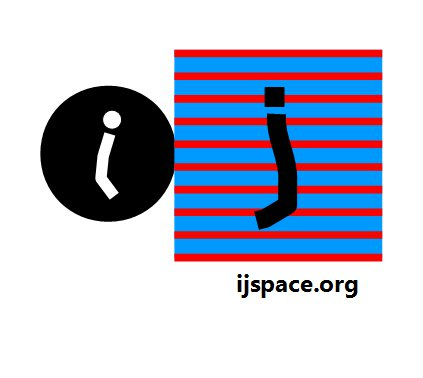# Home

 Why Only Three Dimensions? 12th May 2017 Updated on 19th November 2018 “ In what way does it become manifest in the fundamental laws of physics that space has three dimensions?" - Paul Ehrenfest, KNAW, Proceedings, 20 I, 1918, Amsterdam, 1918, pp. 200-209,  Reference: www.pbs.org     "I shall therefore content myself with the statement that if the student, in solving these equations, does not fail to make use of division wherever possible, he will surely reach the simplest terms to which the problem can be reduced. " - René Descartes in La Géometrié A basic fact of life which seems to puzzle us, is that we are restricted in a three dimensional world.  It would be nice to be able to become higher dimension beings and to be able to do things no mortal can do.  It is actually more of a fantasy when we allow matter over mind.  Mind is known to have no dimensional constraints as long as the knowledge exists.  Without this knowledge we are no better than a dead log with multiple physical dimensions, as useless in one dimension as in many.  In fact the inner workings of mind can not be precisely described by theories and equations.     Various explanations for the existence in a physical three dimensional universe, are based on string theory, the requirements of thermodynamics, the experiments performed at CERN and so on1.  Our objective here is not to summarize these theories.  However we will like to point out that all these ideas are trying to explain a reality which is measurement based, measurements which themselves are limited by the observer's capacity to make them.  The observer's capacity is infinitesimal when it comes to measuring an infinite source.  We will like to show that the existence in three dimension should have a phenomenological explanation.     Let us reintroduce the following diagram used in the previous blog describing an infinite source.  We will be discussing q = 3 information-state which essentially is our universe, whose measurement is based on the electron-photon interaction.  We will argue that as  macroscopic observers ObsM's we have no option but to exist in 3-D space.  More importantly the same is true for the macroscopic observers in q = 2 and q = 1 information-states too.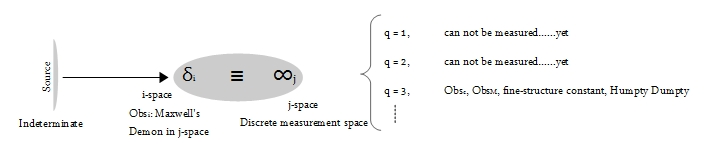While developing the physical description in discrete measurement space or j-space, we ran into the problem of the precise measurement of the origin.  We used anharmonic coordinates combined with Pauli's Spin matrices to develop a geometrical structure with central-force nature, which had to followed by the any measurements made by ObsM.  The examples were gravitational and electrostatic forces.  In this description, the physical reality i.e. the universe existed in Λ∞ plane.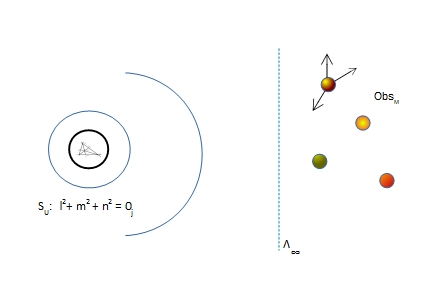In the diagram shown above, various structures being measured by ObsM exist in Λ∞ plane.   The measurements by ObsM on these structures, will follow the central-force nature.  An example is our own earth with the organic mechanisms behaving as macroscopic observers.  The positions of normal vectors as measured by the observer in the Euclidean space on one of the observers, is shown as reference.  The normal vectors are drawn with respect to the physical origin of the structure in Euclidean space.  We need to understand the correlation between the normal vectors in the Euclidean space of ObsM to the unit-point sphere SU.     We note that SU was the result of the application of Spin Matrices and therefore we were allowed to develop the description in the complex-space2.  We can assume that SU is represented by an isotropic vector in the discrete measurement space.  Therefore its fundamental form3 is equal to zero or in other words its value is less than 0j2,  where 0j2 is the smallest value which can be possibly measured by a measurement-system, whose design is based on the electron-photon interaction or QED space.  Thus the observer ObsM in Λ∞-plane will always measure location of SU as 'zero' irrespective of the structure ObsM is on and the location at which ObsM is on, on a given structure.       Consequently our description in j-space can be simplified to assume that respective normals for all the structures in Euclidean space, are drawn with respect to SU.  Or all the structures in Λ∞-plane have the unit-point sphere SU as their origin as shown below.  Thus we have translated the limited capacity of the observer to measure a source into a geometrical context in Euclidean space, where all the structures in Λ∞-plane irrespective of the distance between them or their respective existence on different instances on the time scale, are measured with respect to the unit-point sphere SU.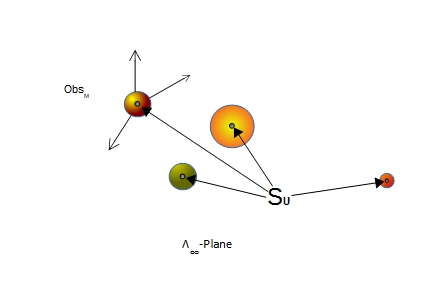Essentially by introducing SU as origin or the point of reference, we have transformed the physical space or universe as we know it, into Λ∞-sphere around SU, as shown in the diagram below.  Next we have to worry about time variable.  We note that time will exist only in Λ∞-plane or outside Λ∞-sphere, as the time is an entropy driven characteristics associated with observer ObsM which can measure structures only in Λ∞-plane.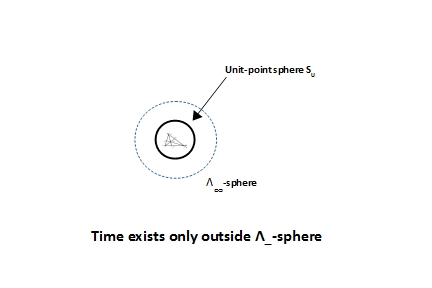Whatever classical structure formation takes place as a consequence of measurements, it must be time-dependent and hence it is restricted to the region outside Λ∞-sphere.  We also note that the information content decreases as we move away from SU4.  An structure which is allowed to progress (equivalently the growth of organics), must be able to measure the information space precisely to proceed.  This is possible, only if the observer associated with the growing structure under consideration, measures the information-space in the region outside Λ∞-sphere, moving outwardly away from the unit-point sphere SU.  (For example if the observer was to measure physical structures inside Λ∞-sphere, while moving inwards in the direction of SU, the observer's capacity must increase with respect to time. This is a contradiction since the entropy inherent in j-space dictates that the observer's measurement capacity must always go down, with the progression along the time axis.)      The unit-point sphere SU essentially is a topological structure 5, impenetrable and immeasurable by the macroscopic observer ObsM.  Whatever information ObsM receives based on its measurement is a small subset of the information available on the surface of SU.  Only ObsC with (v/c =1 ) capacity can measure all the information available on the surface of SU.      The fact that SU is spherical, forces the 3-D constraint on the physical space as the growth or the progress in physical space, is allowed only when moving away from SU on Λ∞-sphere.  It does not matter if the value of q is 3, 2, or 1.  In each information space there will be three dimensions, accompanied by an entropy driven irreversible time-axis specific to the q-value.  The macroscopic observers in q = 1 and q = 2 states will also be restricted to three physical dimensions with their own respective time axes.  The information contained in single unit of length and time will be however vastly different between q = 1, q = 2 and q = 3 states.  The existence in 3-D, thus remains an undisputed reality in discrete measurement space we have called j-space.  It is also worthwhile to mention that the existence in 3-D and time-axis are mutually dependent on each other.  Hence 3-D space automatically implies existence of 4-D time-space, i.e (time, x, y, z).     We have to differentiate between the actual reality and the description provided by the mathematics.  The use of probability in quantum mechanics does not make the physical reality random.  It merely provides us with better estimates of physical measurements performed at very low dimensions, at the same time requiring extraordinary amount of resources in coordinate frame of reference of ObsM.  We can explain some of the facts known to us using quantum mechanics, but not all with required accuracy and clarity.  In fact we do not know anything at all about what we really do not know.     Similarly a higher dimension space, is a sophisticated mathematical construct to provide a better understanding of an indeterminate situation.  The physical dimensions of more than three, will not exist in q = 1 and q = 2 information states either.  However an observer from q = 3 information state may use higher dimension algebra such as tensors to describe the q = 1 and q = 2 information states.  Similarly an observer from q = 2 information state may use mathematical constructs with dimensions higher than three, to describe the q = 1 information space6.       In determining the requirements for the restrictions on the physical dimensions, we have used only the phenomenological facts, such as an infinite source and unit-point sphere, which came out while deriving the value for the fine structure constant.  It will be worthwhile to note that we have only discussed the existence in 3-D, for which we have used structures of spherical shapes as an approximation.  We have not discussed the stability of theses structures.      The existence does not automatically translate into the stability.  The stability of physical structures in j-space is based on the formation of least-energy surfaces, which are more likely to be ellipsoids than spheres.  The formation of least-energy surfaces for the respective stability of the physical structures, applies to all states in j-space irrespective of their q-values (q = 1, q = 2, q = 3 and so on ).  It represents a very important fundamental requirement in discrete measurement space, which we shall discuss some other time.   ------------------------------------------------------- 1. "How Many Dimensions Does the Universe Really Have?" by Paul Halpern,  http://www.pbs.org/wgbh/nova/blogs/physics/2014/04/how-many-dimensions-does-the-universe-really-have/ 2. In discrete measurement space we can not take the existence of complex numbers for granted.  We have to justify using them by providing appropriate context, such as nature of space described by spinors in relativistic limits. 3. The fundamental form of a vector X in n-dimension is defined as Φx = x12+ x22+..............+ xn2.  For an isotropic vector in j-space, the individual component may have negative values and combined together the value of  Φx comes out to be below 0j2, the threshold of the measurement capacity in j-space.  Other possibility is that the contribution of each component even though positive when summed up, is still below 0j2(which is a finite number in j-space). 4. The motion towards SU is allowed within an infinitesimal limit, iff the observer has quantum capacity. 5. The spherical shape of this topological structure is the result of the constraints imposed by the complex-space where i-axis and j-axis are perpendicular to each other.  This orthogonality between real and imaginary axes, is an assumption which is not supported by the discrete measurement space.  Conceptually SU can be of any form or shape unaffected by time. 6.  We keep in mind what we stated before, a basic principle without proof as: "Every observer moves towards the state with maximum information, the observer can measure."  Please note the emphasis on "can measure".  If a state with infinite information is available but the observer can not measure it then the observer can not move towards it, however the observer will stay in orbit.  Gravity is not necessarily the only force we may have to worry about. Previous Blogs:   Infinite Source Nutshell-2016 Quanta-II Quanta-I EPR Paradox-II   EPR Paradox-I    Nutshell-2015